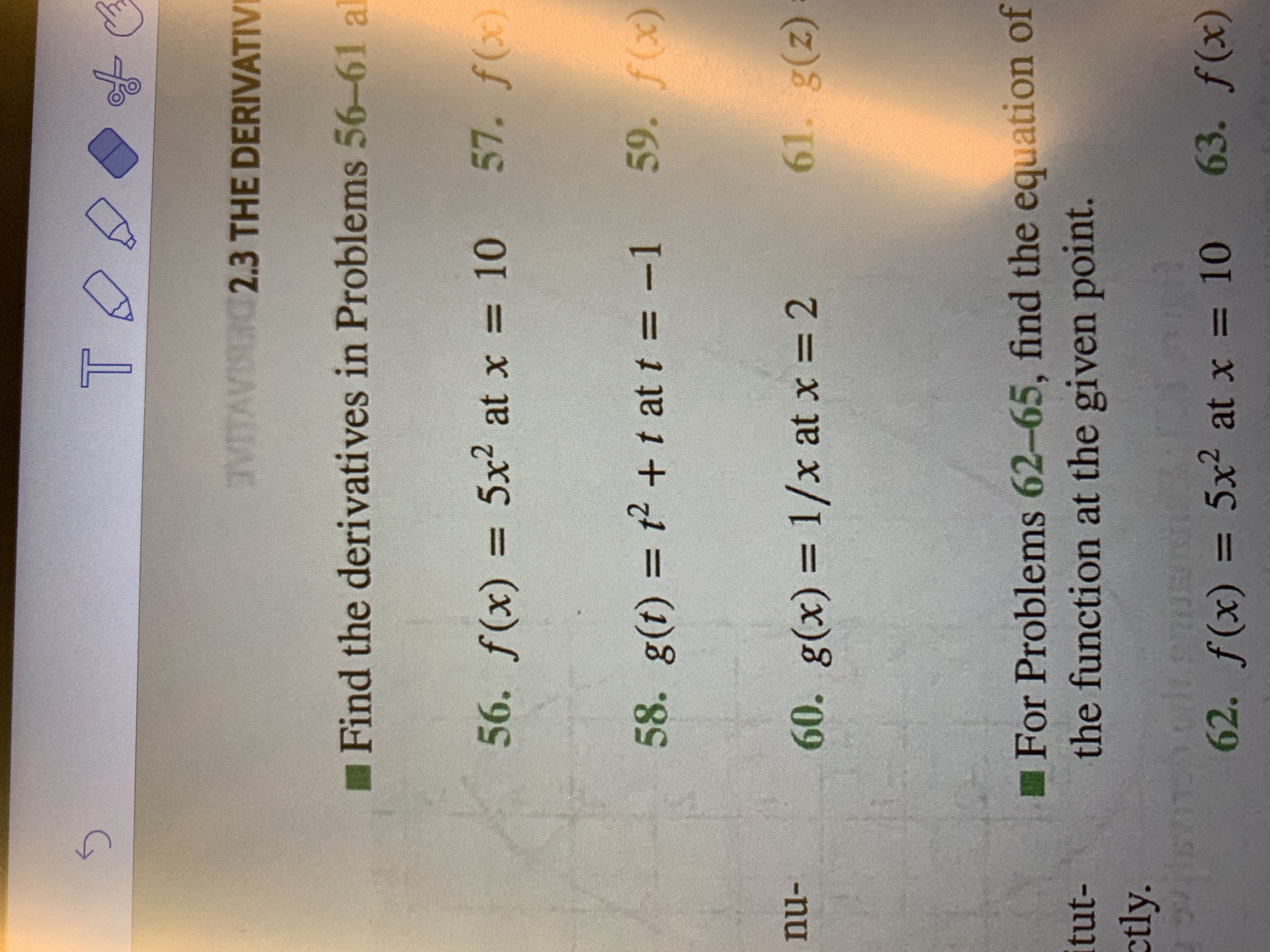# T VTAIG23 THE DERIVATIV Find the derivatives in Problems 56-61 a 57. f(x) 10 56. f(x)= 5x2 at x 59. f(x) 58. g(t) 2 + t at t = -1 61. g(z) nu- 60. g(x) 1/x at x 2 For Problems 62-65, find the equation of the function at the given point. itut- etly. eh orlt emusn 62. f(x) 5x2 at x 63. f(x) 10

Question

58.help_outlineImage TranscriptioncloseT VTAIG23 THE DERIVATIV Find the derivatives in Problems 56-61 a 57. f(x) 10 56. f(x)= 5x2 at x 59. f(x) 58. g(t) 2 + t at t = -1 61. g(z) nu- 60. g(x) 1/x at x 2 For Problems 62-65, find the equation of the function at the given point. itut- etly. eh orlt emusn 62. f(x) 5x2 at x 63. f(x) 10 fullscreen

### Want to see this answer and more?

Experts are waiting 24/7 to provide step-by-step solutions in as fast as 30 minutes!*

*Response times vary by subject and question complexity. Median response time is 34 minutes and may be longer for new subjects.
Tagged in
MathCalculus

### Derivative